Courses

# Zeroes and Poles in Complex Analysis - Mathematical Methods of Physics, UGC - NET Physics Physics Notes | EduRev

## Physics : Zeroes and Poles in Complex Analysis - Mathematical Methods of Physics, UGC - NET Physics Physics Notes | EduRev

The document Zeroes and Poles in Complex Analysis - Mathematical Methods of Physics, UGC - NET Physics Physics Notes | EduRev is a part of the Physics Course Physics for IIT JAM, UGC - NET, CSIR NET.
All you need of Physics at this link: Physics

In mathematics, a zero of a function f(x) is a value a such that f(a) = 0.

In complex analysis, zeros of holomorphic functions and meromorphic functions play a particularly important role because of the duality between zeros and poles.

A function f of a complex variable z is meromorphic in the neighbourhood of a point z0 ) if either f or its reciprocal function 1/f is holomorphic in some neighbourhood of z0 ) (that is, if f or 1/f is differentiable in a neighbourhood of z0). If z0 is zero of 1/f , then it is a pole of f.

Thus a pole is a certain type of singularity of a function, nearby which the function behaves relatively regularly, in contrast to essential singularities, such as 0 for the logarithm function, and branch points, such as 0 for the complex square root function.

Definitions

A function of a complex variable z is holomorphic in an open domain U if it is differentiable with respect to z at every point of U. Equivalently, it is holomorphic if it is analytic, that is, if its Taylor series exists at every point of U, and converges to the function in some neighbourhood of the point. A function is meromorphic in U if every point of U has a neighbourhood such that either f or 1/f is holomorphic in it.

zero of a meromorphic function f is a complex number z such that f(z) = 0. A pole of f is a zero of 1/f.

If f is a function that is meromorphic in a neighbourhood of a point z0 of the complex plane, then there exists an integer n such that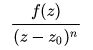is holomorphic and nonzero in a neighbourhood of z0 (this is a consequence of the analytic property). If n > 0, then z0 is a zero of order (or multiplicity) n. Sometimes the term order of vanishing is interchangeably used. If n < 0, then z0 is a pole of order |n|. Simple zero and simple pole are terms used for zeroes and poles of order |n| = 0. Degree is sometimes used synonymously to order.

This characterization of zeros and poles implies that zeros and poles are isolated, that is, every zero or pole has a neighbourhood that does not contain any other zero and pole.

Because of the order of zeros and poles being defined as a non-negative number n and the symmetry between them, it is often useful to consider a pole of order n as a zero of order –n and a zero of order n as a pole of order –n. In this case a point that is neither a pole nor a zero is viewed as a pole (or zero) of order 0.

A meromorphic function may have infinitely many zeros and poles. This is the case for the Gamma function (see the image in the info box), which is meromorphic in the whole complex plane, and has a simple pole at every non-positive integer. The Riemann zeta function is also meromorphic in the whole complex plane, with a single pole of order 1 at z = 1. It has no zeros in the left halfplane besides at all even integers along the negative real line, and the Riemann hypothesis is the conjecture that all other zeros are along Re(z) = 1/2.

In a neighbourhood of a point z0 a nonzero meromorphic function f is the sum of a Laurent series with at most finite principal part (the terms with negative index values):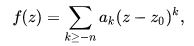where n is an integer, and  a - n ≠ 0 Again, if n > 0 (the sum starts with   a - |n| (z - z0)-|n|, the principal part has n terms), one has a pole of order n, and if n ≤ 0 (the sum starts with a - |n| (z - z0)-|n|,, there is no principal part), one has a zero of order |n|.

At infinity

A function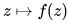is meromorphic at infinity if it is meromorphic in some neighbourhood of infinity (that is outside some disk), and there is an integer n such that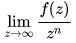exists and is a nonzero complex number.

In this case, the point at infinity is a pole of order n if n > 0, and a zero of order n if n < 0.

For example, a polynomial of degree n has a pole of degree n at infinity.

The complex plane extended by a point at infinity is called the Riemann sphere.

If f is a function that is meromorphic on the whole Riemann sphere, then it has a finite number of zeros and poles, and the sum of the orders of its poles equals the sum of the orders of its zeros.

Every rational function is meromorphic on the whole Riemann sphere, and, in this case, the sum of orders of the zeros or of the poles is the maximum of the degrees of the numerator and the denominator.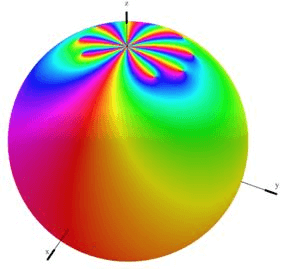Examples

• The function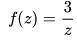is meromorphic on the whole Riemann sphere. It has a pole of order 1 or simple pole at z = 0, and a simple zero at infinity.

• The function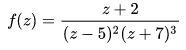is meromorphic on the whole Riemann sphere. It has a pole of order 2 at z = 5, and a pole of order 3 at z = -7,. It has a simple zero at z = -2, and a quadruple zero at infinity.

• The function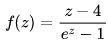is meromorphic in the whole complex plane, but not at infinity. It has poles of order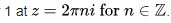. This can be seen by writing the Taylor series of earound the origin.

• The function

f (z) = z

has a single pole at infinity of order 1, and a single zero at the origin.

All above examples except for the third are rational functions. For a general discussion of zeros and poles of such functions, see Pole–zero plot § Continuous-time systems.

Function on a curve

The concept of zeros and poles extends naturally to functions on a complex curve, that is complex analytic manifold of dimension one (over the complex numbers). The simplest examples of such curves are the complex plane and the Riemann surface. This extension is done by transferring structures and properties through charts, which are analytic isomorphisms.

More precisely, let f be a function from a complex curve M to the complex numbers. This function is holomorphic (resp. meromorphic) in a neighbourhood of a point z of M if there is a chart φ such that  f o φ-1 is holomorphic (resp. meromorphic) in a neighbourhood of φ (z) Then, z is a pole or a zero of order n if the same is true forφ (z).

If the curve is compact, and the function f is meromorphic on the whole curve, then the number of zeros and poles is finite, and the sum of the orders of the poles equals the sum of the orders of the zeros. This is one of the basic facts that are involved in Riemann–Roch theorem.

Offer running on EduRev: Apply code STAYHOME200 to get INR 200 off on our premium plan EduRev Infinity!

159 docs

,

,

,

,

,

,

,

,

,

,

,

,

,

,

,

,

,

,

,

,

,

,

,

,

;